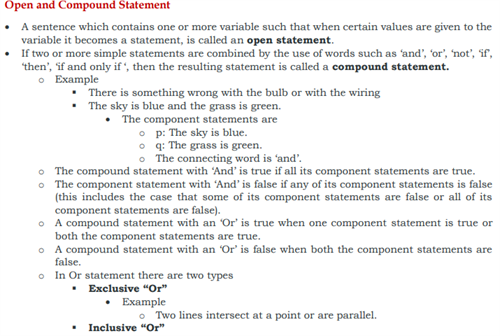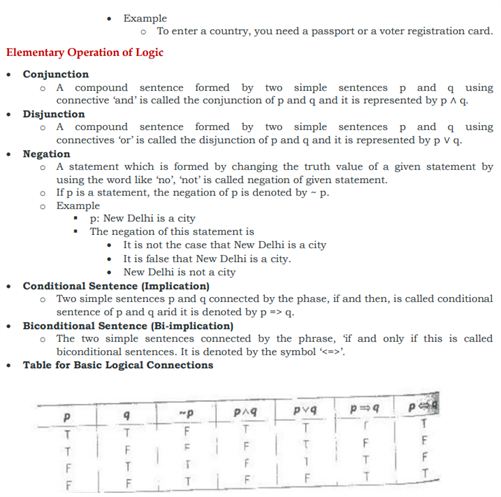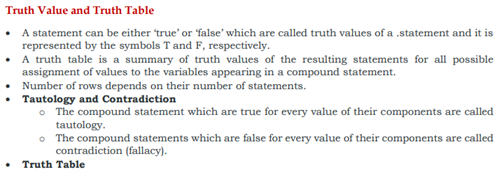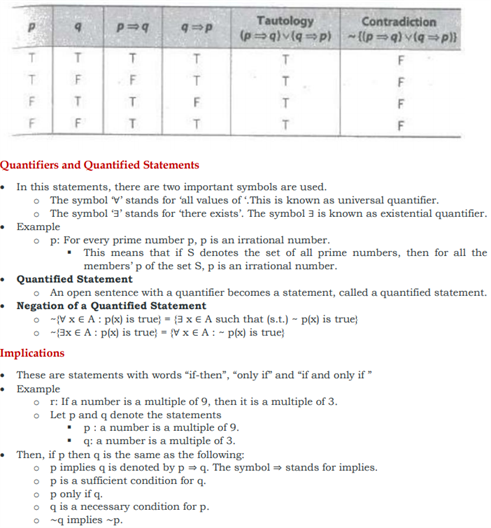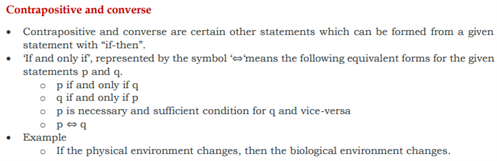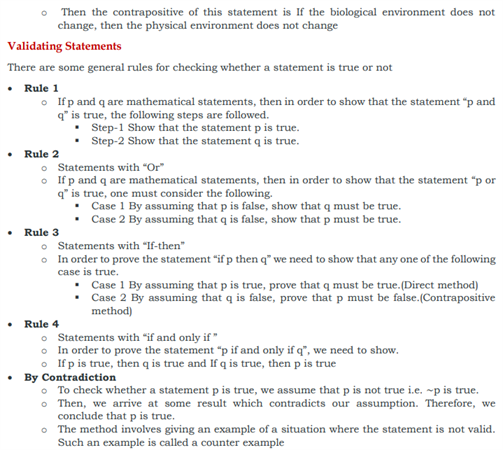# Revision Notes For CBSE Class 11 Maths Chapter 14 Mathematical Reasoning

Class 11 Maths Revision Notes Chapter 14 Mathematical Reasoning comprises of all the basic fundamentals of this chapter. These notes are must to have while preparing for anything related to this chapter, whether it could be an exam or casual practicing. For your ease, we have mentioned some definition:

• In mathematical language, there are two kinds of reasoning – inductive and deductive Logic
• Logic is the subject that deals with the method of reasoning. It provides us with rules for determining the validity of a given argument in proving the theorem.

Statement (Proposition)

• The basic unit involved in mathematical reasoning is a mathematical statement
• A statement is an assertive sentence which is either true or false but not both a true statement is called a valid statement. Otherwise, it is called an invalid statement.
• Statements are denoted by the small letters i.e., p, q, r … etc.

Example

• Mathematics is fun.
• This sentence is subjective in the sense that for those who like mathematics, it may be fun but for others, it may not be. This means that this sentence is not always true. Hence it is not a statement.
• Statement should be a “mathematically acceptable” statement
• Ambiguous sentence is not acceptable as a statement in mathematics.

The notes of this chapter will enlighten students with the open and compound statement, the elementary operation of logic, etc. They will find here the definition of various terms such as contrapositive and converse, implications, truth value, and truth table etc.

You can find here the class 11 revision notes for maths chapter 14 mathematical reasoning.